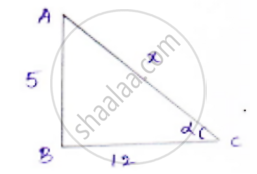Share

# In the Following, One of the Six Trigonometric Ratios is Given. Find the Values of the Other Trigonometric Ratios. Tan Alpha = 5/12 - CBSE Class 10 - Mathematics

#### Question

In the following, one of the six trigonometric ratios is given. Find the values of the other trigonometric ratios.

tan alpha = 5/12

#### Solution

tan alpha = 5/12

We know that tan alpha ="opposite side/adjacent side"= 5/12

Now consider a right-angled Δle ABCLet x = hypotenuse .By applying Pythagoras theorem

𝐴𝐶2 = 𝐴𝐵2 + 𝐵𝐶2

𝑥2 = 52 + 122

𝑥2 = 25 + 144 = 169

𝑥 = 13

sin α = "adjacent side"/"hypotenuse"= 5/13

cos α =  "adjacent side"/"hypotenuse" = 12/13

cot α = 1/tan alpha = 12/15

cosec α = 1/sin alpha = (1/5)/13 = 13/5

sec α = 1/cos alpha = (1/12)/13 = 13/12

Is there an error in this question or solution?

#### APPEARS IN

Solution In the Following, One of the Six Trigonometric Ratios is Given. Find the Values of the Other Trigonometric Ratios. Tan Alpha = 5/12` Concept: Trigonometric Ratios.
S